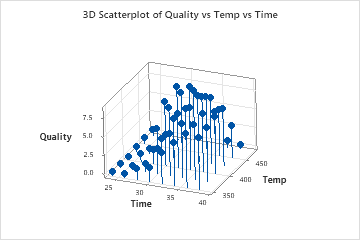# Interpret the key results for 3D Scatterplot

Use a 3D scatterplot to see how a response variable relates to two predictor variables. A 3D scatterplot is a three-dimensional graph that is useful for investigating desirable response values and operating conditions.

A 3D scatterplot contains the following elements:
• Predictor values on the x- and y-axes.
• Response values on the z-axis.
Using the 3D scatterplot, you can view only the actual data values, with no interpolation between the data points such as in a contour plot or 3D surface plot. Use the plot to explore the direction, strength, and linearity of the relationship between the three variables:
• Data points that tend to rise together suggest a positive correlation.
• Data points that tend to rise as other data points tend to decline suggests a negative correlation.
• Outliers fall far from the main group of data points.
###### Tip

Adding project lines helps you visualize each point's position in three-dimensional space. For more information, go to Project lines. Rotate the graph to view the plot from different angles and explore possible relationships in the data. For more information, go to Select display options for 3D Scatterplot and 3D Surface Plot.Key Results: 3D Scatterplot This 3D scatterplot shows the relationship between the time and temperature settings used to cook a frozen dinner and the quality score assigned by food testers. Heating the dinner at shorter time intervals results in under-cooked product and low quality scores. However, heating at the longest intervals combined with the highest temperatures also results in low scores because the food becomes over-cooked. The optimal settings appear to be between 400° and 450° and between approximately 30 and 36 minutes.
By using this site you agree to the use of cookies for analytics and personalized content.  Read our policy